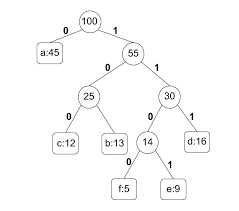# Huffman’s algorithm in Data Structure ?

Sanat Shukla

Total Post:19

Points:135

Posted by  Sanat Shukla
369  View(s)
Ratings:
Rate this:

1.##### Re: Huffman’s algorithm in Data Structure ?

The Huffman’s algorithm is used for creating extended binary trees that have minimum weighted path lengths from the given weights. That makes use of a table that contains the frequency of occurrence for each data element.The Huffman algorithm or Huffman coding is an entropy encoding algorithm.

This is used widely for data compression (like WinZip Compression-WinZip doesn’t use it but!)

The Huffman coding is used in JPEG compression.

The primary idea behind Huffman coding is to encode the most common characters using shorter strings of bits than those used for less common source characters.

That works by creating a binary tree stored in an array.

You also need to know the external path length (sum of all paths from the root to external node) and internal path length (sum of all paths from root to internal node).

Some important  steps for the Huffman Algorithm

• First Step:- Create a leaf node for each character. Attach the character and its weight or frequency of occurrence to the priority queue.
• Second Step: Repeat Steps 3 to 5 while the total number of nodes in the queue is greater than 1
• Third Step: Remove two nodes that have the lowest weight (or highest priority)
• Fourth Step: Create a new internal node by merging these two nodes as children and with weight equal to the sum of the two nodes' weights.
• Fifth Step: Add the newly created node to the queue.

Modified On Sep-07-2019 05:07:07 AM